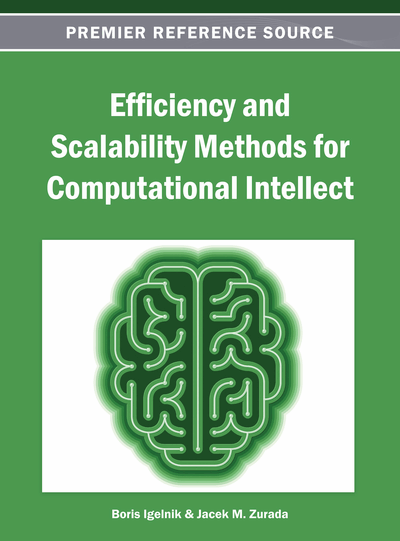# Widely Linear Estimation with Geometric Algebra

Tohru Nitta (National Institute of Advanced Industrial Science and Technology (AIST), Japan)
Copyright: © 2013 |Pages: 16
DOI: 10.4018/978-1-4666-3942-3.ch014
OnDemand PDF Download:
Available
\$37.50
No Current Special Offers

## Abstract

This chapter reviews the widely linear estimation for complex numbers, quaternions, and geometric algebras (or Clifford algebras) and their application examples. It was proved effective mathematically to add , the complex conjugate number of , as an explanatory variable in estimation of complex-valued data in 1995. Thereafter, the technique has been extended to higher-dimensional algebras. The widely linear estimation improves the accuracy and the efficiency of estimation, then expands the scalability of the estimation framework, and is applicable and useful for many fields including neural computing with high-dimensional parameters.
Chapter Preview
Top

## Introduction

Suppose a problem to determine the relationship between two kinds of samples as

and, whereexpresses transposition, obtained from a certain system as that for determining a relationship between a long-term interest rate and a gold bullion price. Assume linear relationship between the two samples, i.e.,

In this case, what is necessary is merely to determine a real-valued coefficient vector

by any means. Here,can be estimated fromusing parameterthus obtained.

Samples,, and parameterare all real values in the above-mentioned problem. However, it is necessary to treat complex-valued data with Fourier transform and wavelet transformation, for example, in fields such as communications, image processing, and speech processing. For cases where numbers other than complex numbers are used, it is also necessary to treat two-dimensional data as one unit.has been used conventionally as an explanatory variable in such cases. It was pointed out recently that there are cases in which sufficient estimates cannot be acquired. Moreover, it was proved effective mathematically to add, the complex conjugate number of, as an explanatory variable. This technique causes the improvement of the accuracy and the efficiency of estimation, then expands the scalability of the estimation framework, and is applicable and useful for neuro-computing. This chapter describes this widely linear estimation for complex numbers, quaternions, and Clifford numbers (Dorst, Fontijne & Mann, 2007).

Top

## Background

First, a technique to treat real-valued data is described. Assume a case in which a true value denoted by a real-valued random variableis estimated from an observed value denoted by a real-valued random vector, whereis the set of real numbers andis a natural number. Then consider an estimated valueexpressed as follows:

(1) where.The problem is to find a parameterthat minimizes mean square error.Such a framework is designated as (real-valued) linear mean square estimation.

## Complete Chapter List

Search this Book:
Reset